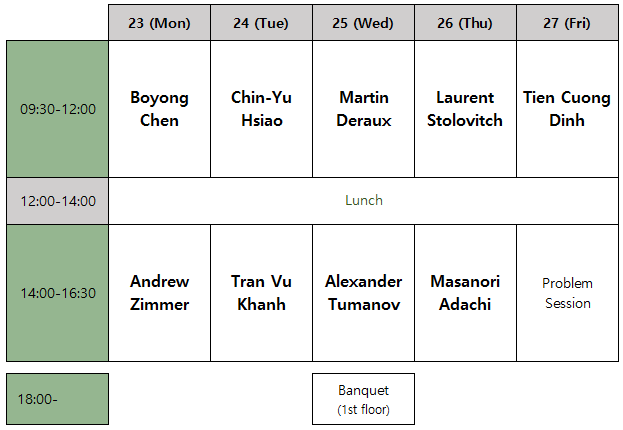﻿ [Math] Progress in Several Complex Variables
 Progress in Several Complex Variables     April 23-27, 2018                                      KIAS 1503
 Program Home > Program- Title: Some aspects of Ohsawa-Takegoshi type theorems

- Abstract: There are few articles in several complex variables have turned out to be as influential and important as Ohsawa-Takegoshi 1987 article. This talk gives a brief introduction of Ohsawa-Takegoshi type extension theorems and their applications. The audience must be warned that the selected contents of the talk is very much infulenced by my own interest, knowledge and limitations. I have not try at all to present a complete picture of the L2 extension theory. That would be a very difficult task.

- Title: Characterizing strongly pseudoconvex domains using their intrinsic geometry
- Abstract: In this talk I will describe some characterizations of strong pseudoconvexity which only use the intrinsic complex geometry of a domain. For instance, we show that for convex domains with \$C^{2,epsilon}\$ boundary strong pseudoconvexity can be characterized in terms of the behavior of the squeezing function near the boundary, the behavior of the holomorphic sectional curvature of the Bergman metric near the boundary, or any other reasonable measure of the complex geometry. I will also describe the proofs which rely on estimating the “Lyapunov exponents” of the “geodesic flow” induced by the Kobayashi metric.

- Title: Szegö and Bergman kernels asymptotics in CR and Complex Geometry

- Abstract: The study of Szegö projection on CR manifolds is closely related to many problems in Complex and CR geometry. In this mini course, I will first introduce some classical results about Bergman kernel on complex manifolds and then introduce Boutet de Monvel-Sjöstrand's classical result about Szegö kernel asymptotic expansion on strongly pseudoconvex CR manifolds. We will deduce Tian-Yau-Catlin-Zelditch asymptotic expansion for ample line bundle from Boutet de Monvel-Sjöstrand's result.

- Title: Regularity properties for the D-bar-Neumann problem and the Kohn-Laplace equation

- Abstract: In this mini-course, I will report recent works on regularity properties for the D-bar-Neumann problem and the Kohn-Laplace equation. I will focus on sufficient conditions called the “potential theoretical conditions” for the L^2-exisitence, global regularity, local regularity, L^p and Holder regularity properties for the solution operators of these problems. The relationship between geometric curvature conditions and potential theoretical conditions will be discussed.

- Title: Discrete groups of automorphisms of the ball

- Abstract: I will explain basic properties of discrete subgroups of automorphisms of the complex n-ball (limit set, domain of discontinuity, manifold at  infinity). I will focus mostly on the case n=2, and describe families of examples, some related to recent developments on real hyperbolic manifolds admitting spherical CR uniformizations (joint work of the speaker with Falbel).

- Title: Complex discs and their applications

- Abstract: A complex (or J-complex) disc is a holomorphic mapping of the standard unit disc to a complex (or almost complex) manifold. We will describe methods for constructing complex discs and discuss their applications. In the first part of the lecture we will discuss applications to analytic continuation of holomorphic an CR functions. In the second part we will consider applications to symplectic geometry and non-squeezing properties of differential equations.

- Title: Introduction Cauchy-Riemann singularities and associated complex dynamics

- Abstract: We shall study the local holomorphic classification of real analytic submanifolds of the complex euclidean space near a point. We shall focus on those which have a  singularity of the Cauchy-Riemann structure :  at each point, the tangent space contains a complex subspace of maximal dimension. A point is called a singularity when this dimension is not constant in  any neighborhhod of it.  Following the pionnering work of Moser and Webster, we associate to such a submanifold a family of germs of holomorphic involutions the holomorphic classification of which is essentially equivalent to the holomorphic classification of the submanifold. By understanding the dynamical/geometrical properties of these involutions, we give a sufficient condition that ensure that one can holomorphically transforms such a manifold into a normal form.  This allow us to  describe, for instance, its local hull of holomorphy. This is a joint work with Xianghong Gong.

- Title: Weighted Bergman spaces of domains with Levi-flat boundary

- Abstract: I will explain an explicit construction of holomorphic functions on the bidisk which are invariant under the diagonal action of certain Fuchsian groups. The quotient of the bidisk by this action is realized as a domain with Levi-flat boundary, on which, in general, the existence of holomorphic functions with slow growth is highly non-trivial. Our construction shows the infinite dimensionality of weighted Bergman spaces of all order in this special case.